## General Mechanical

•xiaofuli
Subscriber

I am doing a simulation of the nonlinear frequency response of a flexible PCB with frequency sweep on ANSYS workbench, there is a problem that no matter what boundary condition(fixed support with acceleration or fixed rotation with displacement) I give it to the PCB, the result is not displaying the same phenomenon as the experiment data. There is a external excitation that will be transferred from MATLAB to the data acquisition and then to the amplifier and finally to the shaker. The most important nonlinearity of the PCB will be from the geometry and the material, as far as I know, if I turn on the large deflection, there will be nonlinear geometry and the structure will be stiffened. besides I try it with the full transient structural analysis for a very long time but still could not finish it. is there anyone could give me some suggestion or help? Thanks very much.

•peteroznewman
Subscriber

Hello Xiaofuli,

I have seen your video and I believe your first model has an overly stiff connection of the IC to the PCB. I think if you separate the IC from the PCB and model more of the detail of the physical connection between the IC and the PCB, the model will have more flexibility and the results will have more flapping of the PCB on the sides.  The illustration below shows a typical DIP package mounted to a PCB.  I don't know what your actual IC connection is to the PCB, this is just an example.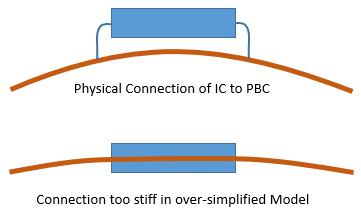It was useful to have a very simple model to get the basic model working. Now you can create a higher fidelity model, but you will pay the price in solution time.

Regards,

Peter

•xiaofuli
Subscriber

Very sorry that this issue had taken you a lot of time But still very thankful for your help.

1. If I neglect the contact nonlinearity and only consider the nonlinear geometry, do I need to change the model of over-simplified with over stiff stiffness? which means that I consider there will be rigid connection between the IC and the PCB. Will it influence a lot for the nonlinear vibration effect?

2. Last time you sent me an excel file with the constant acceleration, which the displacement of the acceleration will be decreased with the frequency increasing. I take a look at the simulation result which I attach it below, If we flip the graph, we make the time at 10s as the starting point and the time at 0s as the ending point, I found out that this will be exactly the nonlinear vibratory effect that I want. do you have some suggestion about this issue?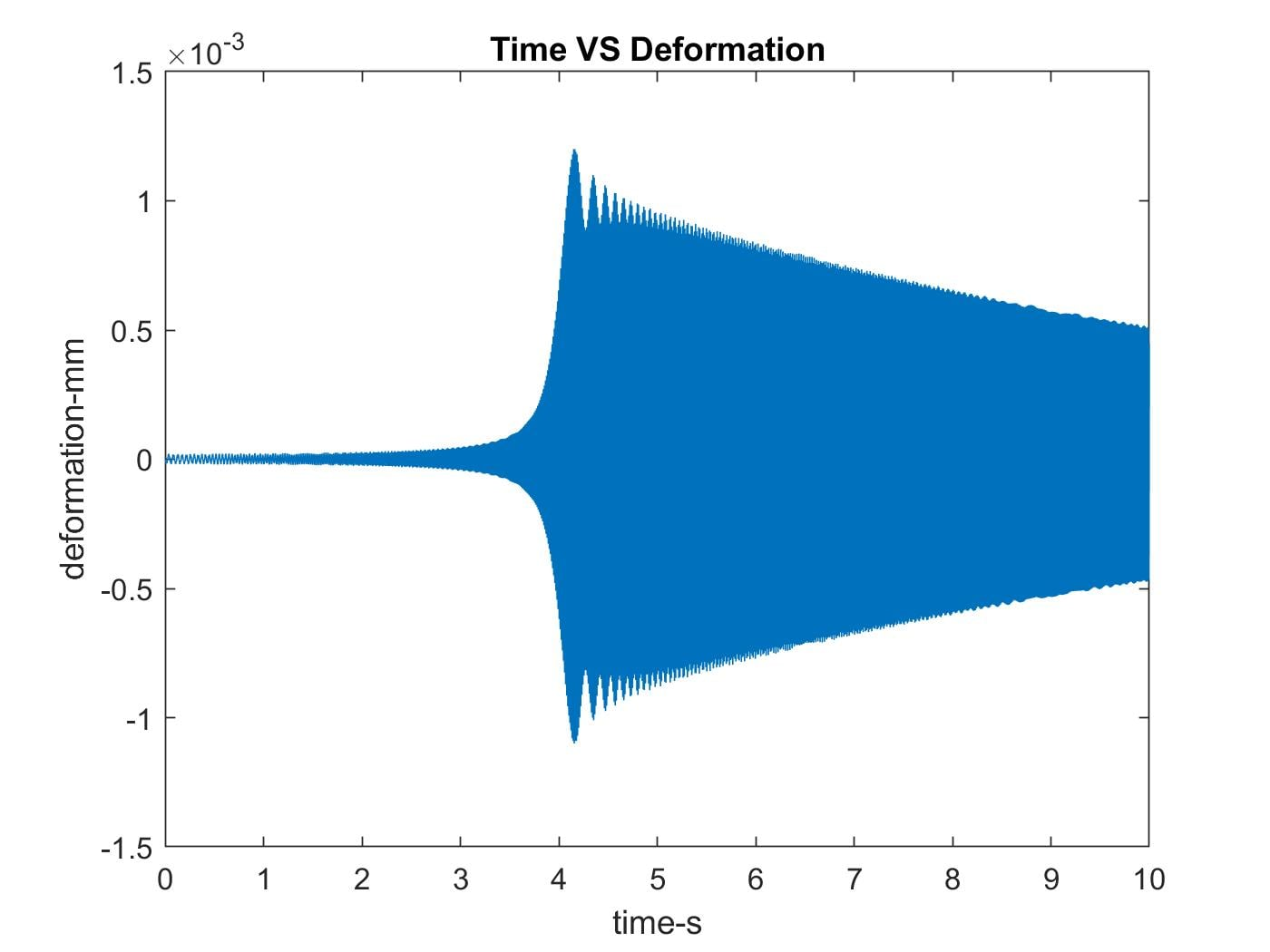Best Regards

xiaofu li

•xiaofuli
Subscriber

1. Do you have some suggestion for the model design on the physical connection between the IC chip and the PCB, Since I was not good at modelling in ANSYS Workbench. Could I make the physical more detailed geometry in SolidWorks? will it work very well for mesh generation within ANSYS?

2. By the way, does the physical connection influence the final result that much? like what I stated earlier, If I do not consider the contact nonlinearity but only the geometry nonlinearity. will it display the nonlinear vibratory effect?

Thanks

Best Regards

•peteroznewman
Subscriber

Today's 1. What does the connection of the IC to the PCB look like?  I showed you an illustration of a Dual-Inline-Package (DIP). that only connects to the PCB along two lines and has flexible legs going up to the package.  There is also a Ball Grid (BGA) which is a different method of connecting an IC to a PCB as shown below, which is much stiffer than the DIP. Please share details of the chip and its connection.

Today's 2. Yes, the physical connection does influence how much the PCB is free to vibrate.

4 days ago 1.   Yes. You need to change the model to get a result more like what you see in the video.

4 days ago 2. You can flip the swept sine wave input and start at the high frequency end and sweep down to the low frequency end.

•xiaofuli
Subscriber

1. I will share more detailed information for the physical connection later on, Since we are making a new PCB now and still are considering the physical connection between the IC and the PCB.

2. Since if I flip the swept sine, things will be different, the up and down frequency sweep will give different nonlinear vibratory result. in fact as for your acceleration input, the down sweep(starting at high frequency) will be the up sweep(start at low frequency) result obtained from the experiment. that is the reason why I feel confused about ANSYS simulation result. The input function will be a displacement function, therefore if the amplitude of the displacement does not change, the acceleration will be increasing all the time in order to keep the same amplitude of the displacement. The attachment file will be the ANSYS Simulation with displacement and fixed rotation, but it seems that the result match good for half of the experiment result, but I did not see the big jump within the simulation result. do you have some suggestion for that? besides I will show you what does the experiment result looks like. Thanks for your help.

Best Regards

Xiaofu Li

•peteroznewman
Subscriber

Xiaofu Li,

I believe you are plotting the displacement of the IC relative to ground (absolute displacement) vs. Time.
I think you should be plotting the displacement of the IC relative to the frame (relative displacement) vs. Time.

It's a mathematical fact that if you sweep with a fixed displacement, the acceleration has to increase with frequency.

Regards,

Peter

•xiaofuli
Subscriber

1. The previous PCB with IC chips at the middle has the connection of the Ball Grid (BGA). Do you have some suggestion that how could I make the BGA connection within ANSYS Workbench of Geometry Modeling?

2. I will try to give the relative displacement with respect to the time. but still have no idea how could I make it in Workbench, do I need to make a remote point? I hope that you could give me some advice for this issue.

Thanks

Best Regards

•peteroznewman
Subscriber

1. How many balls?  What ball diameter?  What ball material? What is the pattern of the BGA?  I think you might build a detailed model of the BGA to characterize the stiffness between the PCB and the IC, then replace the detailed model with a simple bulk solid that has the stiffness properties of the BGA connection in the area where the balls were.

2. You just need a second displacement probe on the fixture. Then you can subtract the fixture probe from the IC probe and you will have the relative displacement.

Regards,

Peter

•xiaofuli
Subscriber

1. Given that we will make a new PCB model, my advisor told me that we will just make the IC chip rigidly glue together with the PCB, there is no special physical connection between them. I thought that we do not need to consider the problem of Dual In-Line or Ball Grid.

2. Since I found out a small issue that If the time span is not large enough for the whole simulation, the Jump-up or Jump-down phenomenon will not happen, but after I change the total time from 10s to 20s. there is a convergence issue happens which said that one of the element have excessive distortion. Could you give me some suggestion for that, I also attach it, besides sometimes if I increase the number of the elements, the problem will not be occurred, but sometimes it does not work. Do you know the reason why?

Best Regards

Xiaofu Li

•peteroznewman
Subscriber

Xiaofu,

You seem to have forgotten the lesson on how many samples are needed to create a good sine wave. The answer is a minimum of 20 samples per period. You created a sine sweep from 80 to 230 Hz over 25 seconds, but you only sampled it at 600 Hz.  600/230 = 2.6 samples per period at 230 Hz. You should have sampled it at 4600 Hz or 20 samples per period.  The sampling frequency is not even adequate at 80 Hz where you have 600/80 = 7.5 samples per period.

Look at the quality of the input you are giving to your model...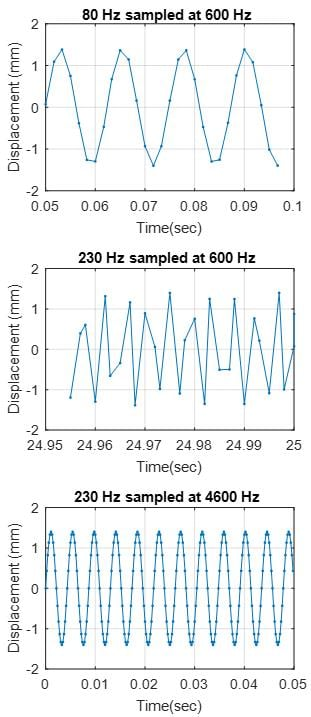The third plot is what your model needs. I know that these large time histories take a long time to load into the model, but you won't get a high quality result if you don't have a high quality input. You may have heard the term "garbage in, garbage out"?

Regards,
Peter

•xiaofuli
Subscriber

1. I do remember the lesson on the 20 sample points for one period, Since if I give 15000 data points, the time step will be 25/15000(s), the reciprocal value of it will be the sampling frequency which is 600Hz, very thankful for that insightful advice. Besides before trying it with 15000 data points, if I give it 8000 data points, it will work well without any error, just the result looks very terrible. Do you know the reason why the case of 8000 data points work but 15000 data points does not work?

2. Does the number of elements for the mesh and the time step influence the convergence? Since from my point of view, If I increase the time steps, there will be less possible that the sharp change of the input will occur, therefore the convergence will be easier to be completed.

Best Regards

Xiaofu Li

•peteroznewman
Subscriber

If you want to  simulate 25 seconds and have a 230 Hz frequency in the input data, you need to sample that signal at 4600 Hz or 115,000 data points in the displacement input for 25 seconds. When you solve, you want a time step of 1/4600 or 2e-4 seconds.

I understand that is a tremendous amount of data. Welcome to nonlinear transient dynamics.  This is why linear analysis methods such as Harmonic Response were developed; you can get a linear solution with a small amount of data. However you require a nonlinear solution due to the large displacements and the stress stiffening that is occurring.

Just because the solver doesn't fail with just 8000 points, doesn't mean that it is a valid result. Have you heard of aliasing?  If you sample a high frequency process at a low sample rate, you can see data that is wrong.  Below is an example of aliasing. The circles show the data that was saved in the results when the sample rate was 8 kHz. From the circles, you see a signal that is 0.5 Hz or a cycle with a period of 2 seconds. But that is totally wrong, the real signal has a frequency of 7.56 kHz, but the sampling rate was only 8 kHz when it should have been sampled at 8*20 or 160 kHz, then you would see the correct result of a 7.56 kHz signal. This example uses higher frequencies than your problem, but it has a nice illustration of aliasing in the plot below.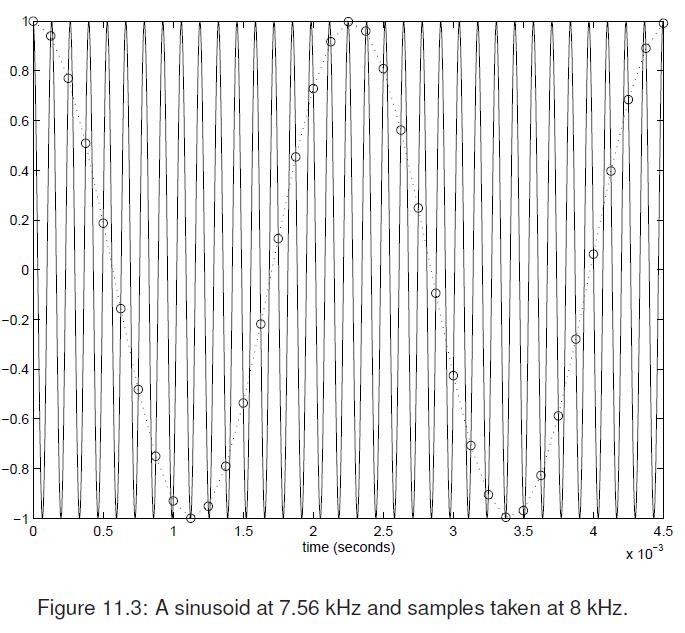2. After you sample the solution output data at an adequate rate, then you can worry about convergence. Fortunately, since the time steps are so small, convergence will not be difficult as long as the input data has an adequate sample rate, and I have shown above that your input data had a sample rate that was too low, causing sharp changes in the input which should not be there.

Kind regards,
Peter

You can show your appreciation by clicking Like below the posts that are helpful.

•xiaofuli
Subscriber

1. you are right, Since if I could give the sampling rate 20 times higher, the fidelity will be sound preserved.  Thanks for that, what about 10 times higher, Since when we are collecting the experimental data, the sampling rate is just 10 times higher. I remembered that there is a class that I took before, it said that your sampling frequency should at least 2.5 times higher. I thought 10 times higher is already good enough(Since I want to shorten the simulation time). Am I correct?

Best Regards

Xiaofu

•peteroznewman
Subscriber

Xiaofu,

Engineers who analyze the frequency content of signals in the frequency domain can get away with a minimum sampling rate of 2.5 times the highest frequency in the signal, but that is because they are only interested in frequency analysis.

Engineers who have to work in the time domain, not the frequency domain, must sample at a minimum sampling rate of 20 times the highest frequency in the signal because they have to reconstruct a time domain signal, not just compute frequency data.

If you want to reduce the burden on your computer, simulate for 5 seconds instead of 25 seconds. That will make everything go 5 times faster!

Kind regards,
Peter

•xiaofuli
Subscriber

1. I do have a question that If I use the 5s instead of 20s, even though everything will go 5 times faster, but what about the results, does it still have the high fidelity? Since the real case of the running time of the experiment is 25s. That is what I am concerning, you could make it less time with enough data points, but the thing is that 5s with 200Hz(suppose it is the highest) and 200*20*5=20000(data points), compared with the 20s with 200Hz(suppose it is the highest) and 200*20*20=80000(data points). Does this two cases give the same results? since it is nonlinear case, I think if the frequency sweep time is not slow or long enough, the nonlinear vibratory effect is very difficult to be noticed or attained. Do you have some suggestion?

2. If I have 2 cases: Case 1: Link the transient structural with the Modal Analysis and running them by mode superposition which is fairly linear. Case 2: Use just the single transient structural but turn off the large deflection and the nonlinear effects. What is the difference between case 1 and case 2?

Thanks

Best Regards

Xiaofu Li

•peteroznewman
Subscriber

1. If you want to sweep from 80 Hz to 200 Hz in 20 seconds, that is 6 Hz/second.  You can break that up into four pieces of 5 seconds each.

•  80 - 110  Hz

• 110 - 140 Hz

• 140 - 170 Hz

• 170 - 200 Hz

That will make each analysis more manageable, but if something interesting happens at one of the breakpoints, you might miss it. You could overlap the ranges slightly to avoid that.  That will give the same result as a single 20 second sweep of 80 - 200 Hz without bogging down the computer excessively.

2. The full model has 290 nodes which creates1068 equations. Using modal superposition involves taking six modes that might be described by 10 coefficients for the shape functions, or 60 equations.  It is a lot faster to do time integration on 60 equations instead of 1068 equations.

•xiaofuli
Subscriber

1. you split the 20 seconds into 4 groups, which means that it takes 5s for 80-110Hz with the sampling frequency 2200Hz and 2200*5=11000 data points, but if I would be interested in the breakpoints, which means that the next group will be 105Hz(can also be less or higher whenever it is less than 110Hz) to 140Hz. Do I understand your point correctly?

2. I try the acceleration with fixed support, the frequency sweep is from 80Hz to 200Hz, I give it 80000 data points, which is exactly 20 times higher during 20s time span, but the thing is that I found out the output is just copying the trend of the input. unlike the last time, you help me to create a constant 5g acceleration within 10s, the result of which looks very promising. Do you know the reason? I also attached the ANSYS file, If you have time, you could run it and take a look at it, The acceleration data I got is just to do twice derivative of the displacement forcing function.

3. If I give the fixed support, but at the same time, I also apply the displacement with only Z direction free to move, will it still work when both of them are input as the boundary conditions?

•peteroznewman
Subscriber

1. Yes, you understand the idea of overlap correctly.

Here is an important table calculated in the Modal Analysis.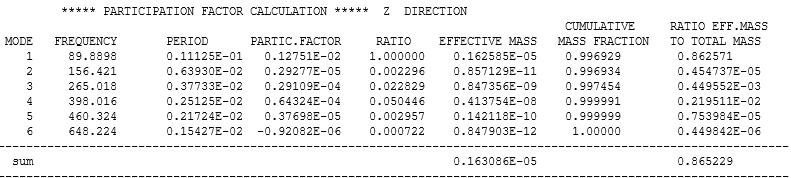It lists the mode frequencies, but also the Effective Mass that is participating in each mode. You can see 99% of the mass moves with mode 1, while only a tiny fraction of the mass moves with any of the higher modes.

2. You didn't say which of the three systems to look at. I looked at system C first, because it was labelled fixed support and acceleration. That has a 10 seconds sweep from 80 - 200 Hz, but is only sampled at 2 kHz or 10 samples per period.  Next I looked at system D, which is labeled Time span 20s. The acceleration-time history in that system is sampled at 3500 Hz. I computed the spectrogram for this acceleration signal. If you intended for this to be 80-200 Hz, you miscalculated. It looks like it tops out at 320 Hz. It is unfortunately sampled below the recommended rate of 20 samples per period.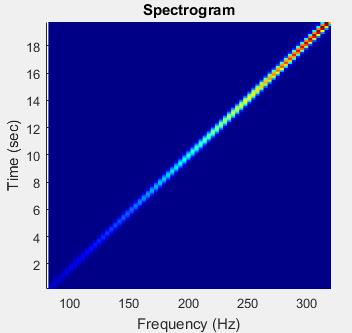I can calculate in seconds the response of a Single Degree-of-Freedom (SDOF) spring-mass-damper system with a specified resonant frequency and Q factor (damping), to that base acceleration input.

If I create a SDOF with a natural frequency of 90 Hz and a Q factor of 10, apply the above acceleration base input, this is the resulting relative displacement: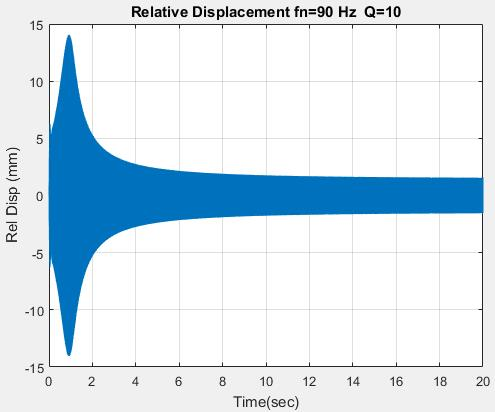You can see that it might be worth starting lower than 80 Hz. Perhaps 60 Hz would be a better starting frequency.

Keep in mind that this is a linear system response. We already established that the nonlinear large displacement and stress stiffening greatly reduces the magnitude of the relative displacement. Note that at 1 second, the input frequency is 90 Hz, which matches the natural frequency of mass-spring-damper system. The ANSYS model has multiple natural frequencies. The first one is around 90 Hz, the second one is 156 Hz.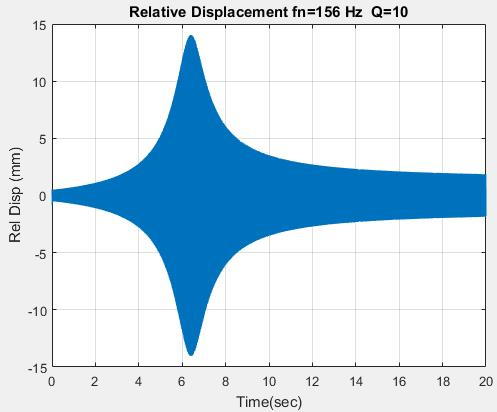I'm running that model to look at the output from ANSYS using the full nonlinear transient solution, but that will have to run overnight before I get results out to 20 seconds, but it made it past 5 seconds so I stopped it to plot some initial results.

Here is the input spectrogram for the acceleration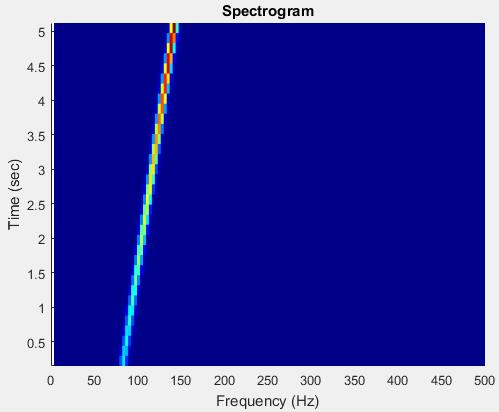Here is the output spectrogram for the Z acceleration of one corner of the IC.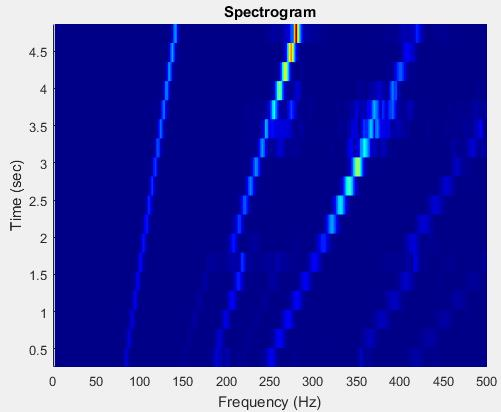While you can see the input frequency in the output, you can see higher frequencies in the output that are excited.

3. You can't have both fixed support and a displacement support on the same face.

Regards,

Peter

•xiaofuli
Subscriber

It might be my mistake to give you the wrong model, I will take a look at my model and get back to you as soon as possible. But very thankful for your help.

1. As you stated "You can see 99% of the mass moves with mode 1, while only a tiny fraction of the mass moves with any of the higher modes." But from the cumulative mass fraction, It seems that even the higher mode, there are almost 99% mass participated into the movement, While from the Ratio Efft.Mass to Total Mass, it seems that as for the mode 1, 86.257%(not 99% as you mentioned) mass will be participated into the move while only a tiny fraction of the mass moves with any of the higher modes.

2. As for the base acceleration, I think you have to use the mode superposition which is a linear solution, Am I correct? If it is the case, then what is the difference between the base acceleration as the input and the acceleration applied to the whole body(As what I did in transient structural with the fixed support and the acceleration) within ANSYS Workbench?

Best Regards

Xiaofu Li

•peteroznewman
Subscriber

1. You can use either column, but the conclusion is the same.

2. Base acceleration means the acceleration signal of the base of a shaker table. You can't put this into ANSYS directly. You can integrate that signal and apply a velocity input, or integrate it again and input a displacement. In the light blue plots above, I am plotting the relative displacement between the base and the mass that is connected to the base through a spring and damper.  If you have a displacement input, which is easy to generate in matlab, you can run that simulation in ANSYS. If you probe the Z displacement of a point on the IC, you have to subtract the Z displacement of the input to the faces fastened to the shaker to get the relative displacement.

Instead of actually moving the base, the acceleration signal can be applied to the mass. This is easy to do in ANSYS and then when you probe the Z displacement of a point on the IC, the base has zero displacement, so you don't have to do the subtraction to get the relative displacement.

I wanted to be able to probe a point on the edge of the PCB and a point on the IC so I went into SpaceClaim and split the body.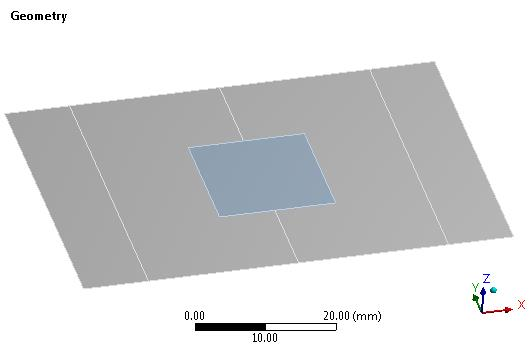Now I can get a probe on the edge of the PCB where you saw large flapping and on the IC at the center of one of its edges.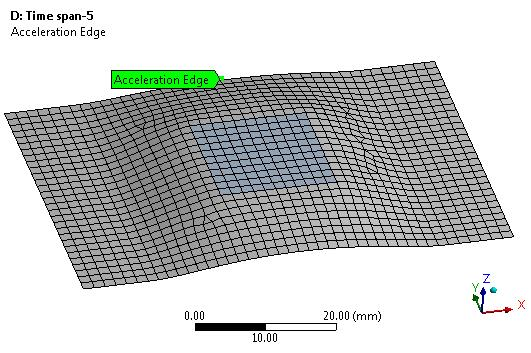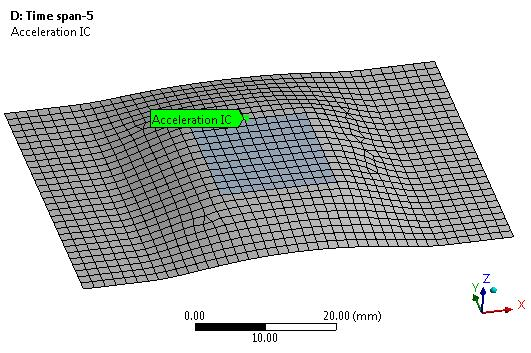I created a 5 second sine sweep from 80 - 190 Hz sampled at 4 kHz. It took 3 hours, 23 min on 8 cores. Here is the input acceleration spectrogram.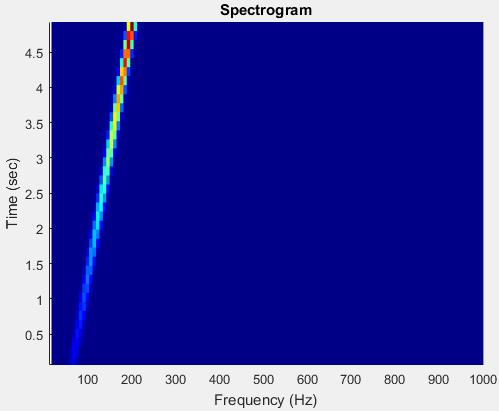I output the data at 4 kHz also.  Below is the acceleration of the IC point.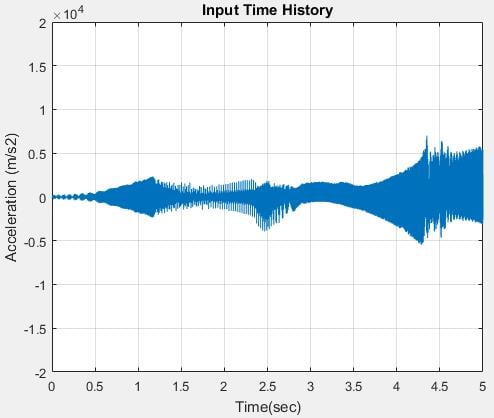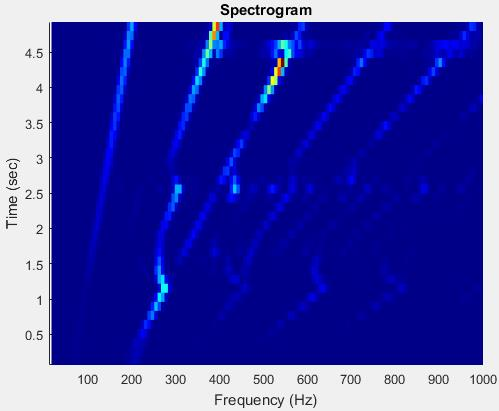Below is the acceleration of the Edge point, note the much higher accelerations occurring on the edge.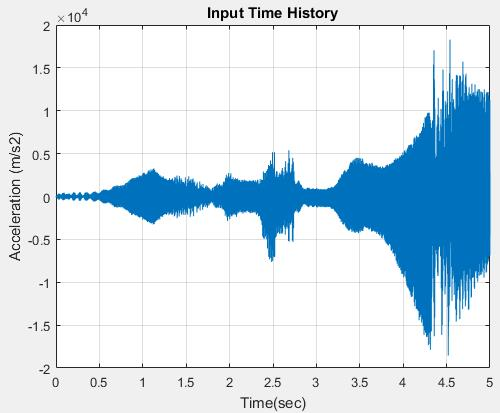You can see the higher frequencies in the response of the edge compared with the IC.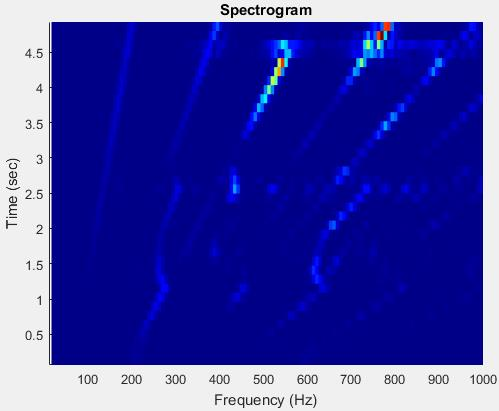Hope this helps.

Regards,

Peter

•xiaofuli
Subscriber

Thanks very much for your help

1. Like what you stated, if I give the displacement input with the fixed rotation at the faces fastened to the shaker within transient structural of ANSYS. Then I need to subtract the input displacement to get the relative displacement. If I am correct, then how could I give the displacement/acceleration signal to the mass. therefore I do not need to subtract the base displacement if I would like to probe the Z-direction response of the IC chip.

Thanks very much, the information you provided is vey helpful.

Best Regards

Xiaofu Li

•xiaofuli
Subscriber

1. I double check the displacement data within the ANSYS, it is just 200Hz instead of the 320Hz as the highest frequency, What kind of MATLAB code did you use, The following is what I use for checking the frequency.

figure
spectrogram(p,kaiser(128,18),120,128,2000,'reassigned','yaxis')

•peteroznewman
Subscriber

Here is a video of my 5 second sweep. Deformation result scale is 5 times larger than true deformation.

This is the last 2.5 seconds at the higher acceleration and frequency of vibration.

The last part is interesting because the pattern changes. I should have cut the length of the video in half again.

•peteroznewman
Subscriber

I set the Options setting in Workbench to show 10 significant digits.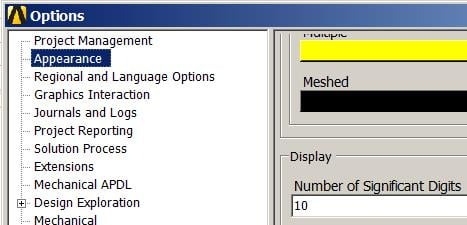I restored the ANSYS_Issues.wbpz file in the zip file above and opened system D, clicked on the Acceleration load and scrolled to the end.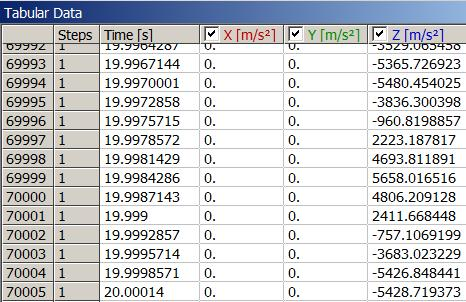If you don't change the displayed digits, and copy cell then paste in matlab or excel, you only get the digits displayed. For example, with the default 5 significant digits displayed, the data look like this: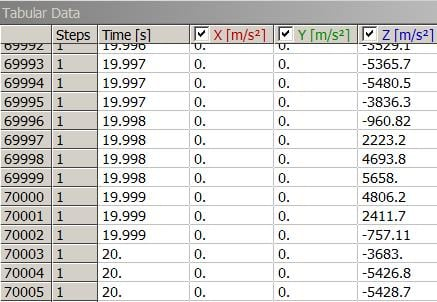Performing numerical analysis on data that lacks the full precision could cause errors.

I took the last 194 rows of acceleration data and reassigned it a new time column starting at zero.
The statistics on that last 0.05 seconds of the 20 second history shows a 317 Hz frequency.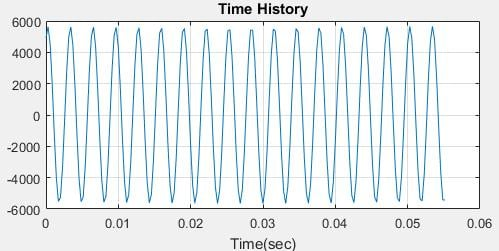I don't know what your matlab code is doing.

Kind regards,

Peter

•peteroznewman
Subscriber

if I give the displacement input with the fixed rotation at the faces fastened to the shaker within transient structural of ANSYS. Then I need to subtract the input displacement to get the relative displacement. If I am correct, then how could I give the displacement/acceleration signal to the mass. therefore I do not need to subtract the base displacement if I would like to probe the Z-direction response of the IC chip.

When you apply a displacement signal to the edges of the PCB clamped to the shaker, all the mass in the IC and PCB are connected to those faces so the stiffness of the PCB transfers the enforced displacement at the clamped edges to all the elemental masses in the structure. You don't give it anything else.

A node at the center of the free edge of the PCB might move differently to a node on the  IC and they both have a different relationship to the motion of the node clamped to the fixture which has the input displacement enforced on it.  When you probe the Z-direction of the node on the free edge, you subtract the Z-direction enforced displacement of the fixture.  Similarly, when you probe the Z-direction of the node on the IC, you subtract the Z-direction enforced displacement of the fixture.  You get two relative displacements: free edge of PCB and IC edge and you must have subtracted the base displacement to get this.

Regards,
Peter

•xiaofuli
Subscriber

1. Thanks again for the digits displaying issues.

2. Does the Solution->Probe->Position means that I could get the displacement response of the IC chip? Since I thought that the directional deformation might not be a good representation of the experiment while the displacement response should be a better choice. If Probe could help me to see what happened for the displacement response. I do meet a problem that shows as following, It seems that I failed to pick the geometry.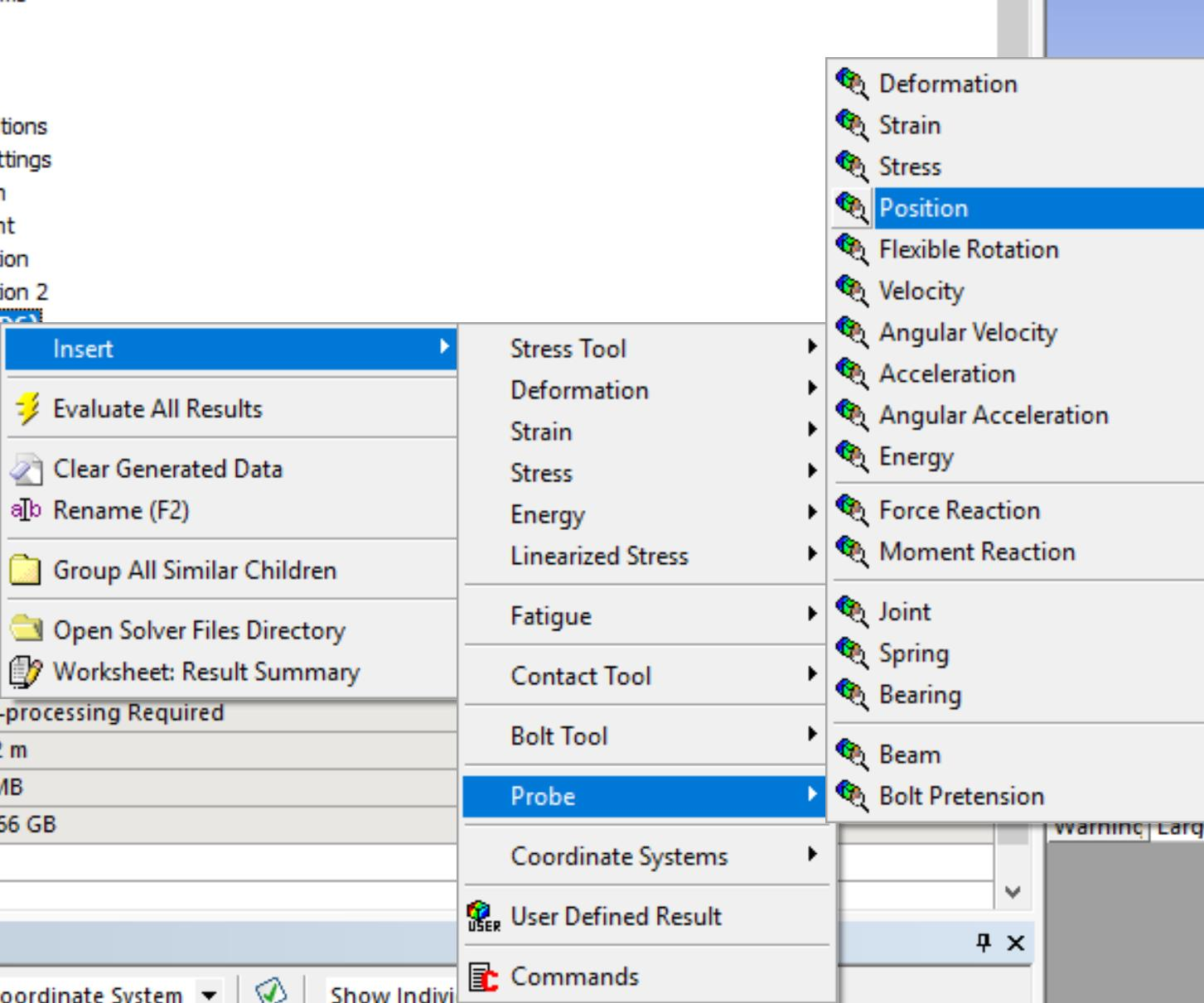3. I would like to know how did you check the frequency by using the spectrogram within the MATLAB? Is that possible that I could take a look at your code, I might use the wrong command.

Best Regards

Thanks

Xiaofu Li

•peteroznewman
Subscriber

2. From the Help system, Position is only for Rigid bodies.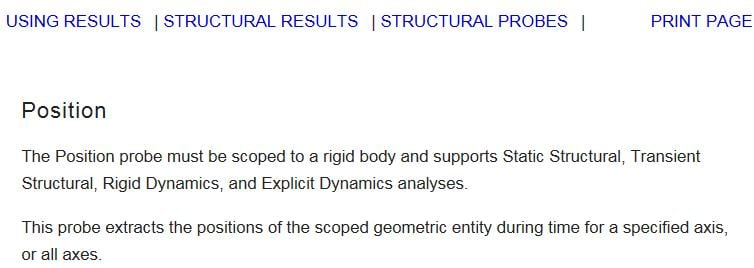3. I use the free matlab vibrationdata GUI package of scripts written by Tom Irvine. You can get a copy of that here, which will let you download a zip file. Extract that into a folder and you will have all the source code.  Add that folder to your matlab path.  Type vibrationdata at the command line and the GUI will start. You will see Waterfall FFT & Spectrogram on the list.  Note that the data has to be in a two-column variable with time in the first column and acceleration in the second column.

Regards,

Peter

•xiaofuli
Subscriber

1. If the probe->position is only for the rigid bodies, then how could I get the displacement response for the IC chip? I think it is a different result from the directional deformation. Like what you mentioned before:"If you probe the Z displacement of a point on the IC, you have to subtract the Z displacement of the input to the faces fastened to the shaker to get the relative displacement." How did you get the Z displacement response? Why do we need to use the relative displacement instead of absolute displacement? If I do not subtract the Z displacement of the input, does it means that I will get the absolute displacement?

2.How did you get the following table from Modal Analysis?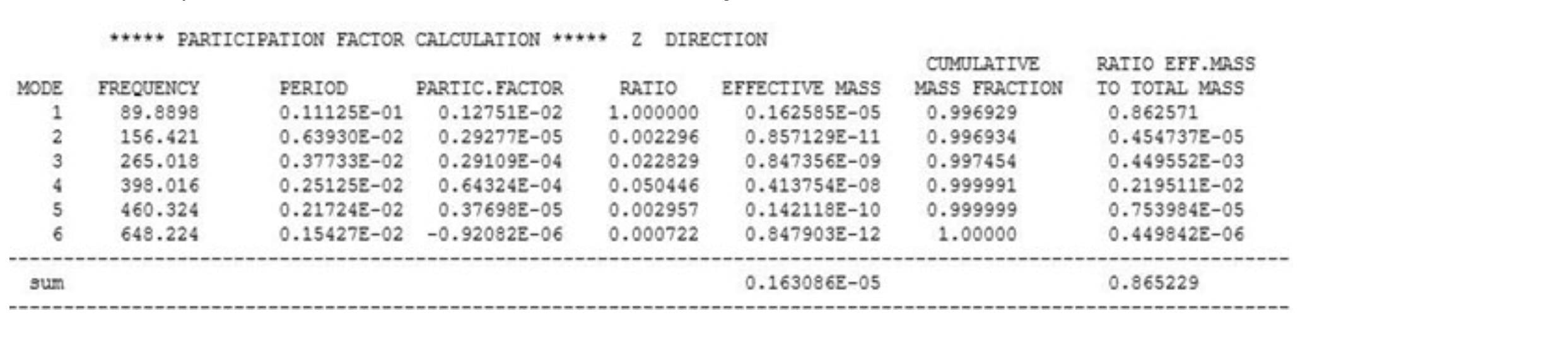Is there any possible solution that we could derive the mass participation within different mode shapes in Nonlinear Case? Since I was also very interested in this part.

Best Regards

Xiaofu Li

•peteroznewman
Subscriber

1. I split the face so that I have a vertex on the free edge of the PCB and a vertex on the side of the IC. Below are the Results for Acceleration, but you would do the same thing for Directional Deformation.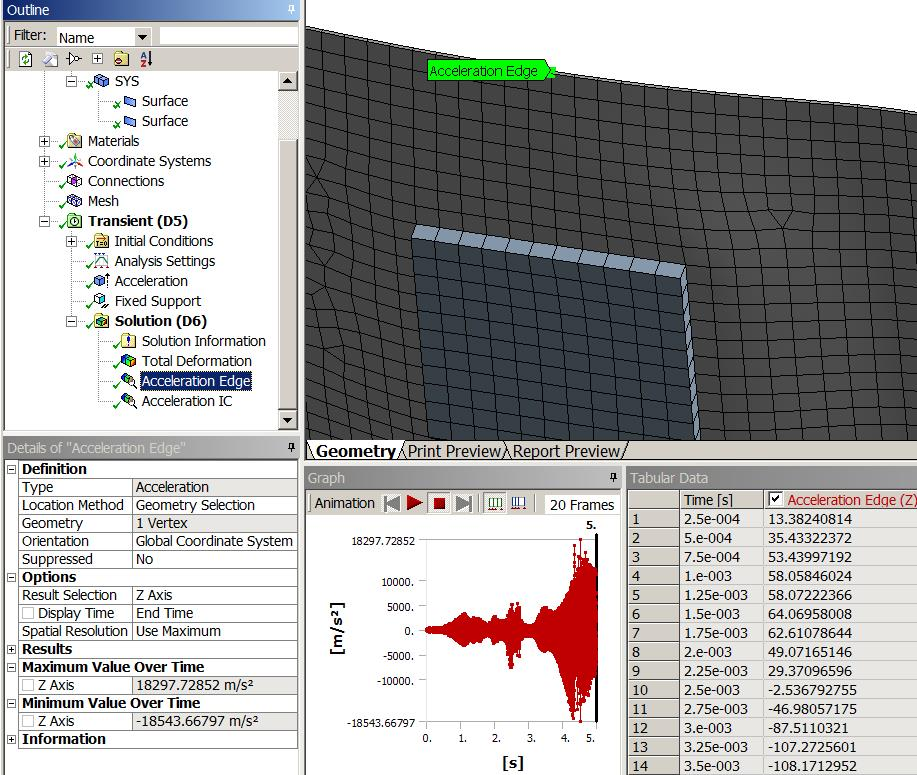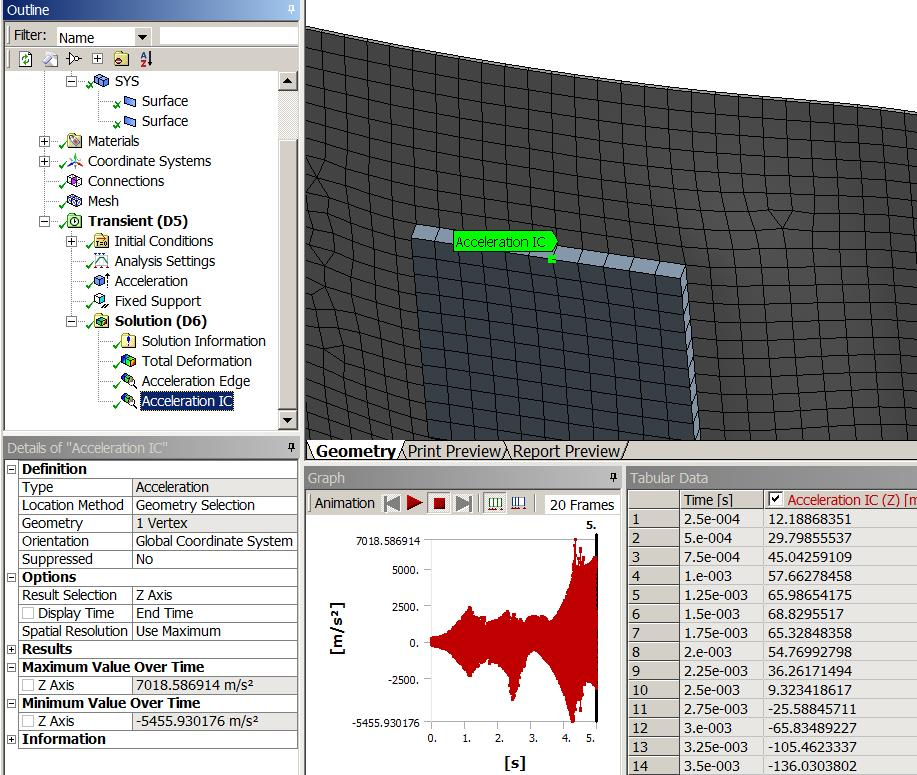Maybe you can make a user defined result to subtract the input displacement from the IC directional deformation result.  I don't know how, I rarely use user defined results. I just used Excel (making sure I had sufficient digits displayed), but I notice that if I select both the input displacement and the output deformation, I can make a Chart with them.

The relative displacement is usually more relevant to vibration results. For example, if you had a sine displacement with an amplitude of 10 mm at 1 Hz, the absolute displacement would be +/- 10 mm, but the relative displacement would be zero since the frequency is so low, the IC just follows where the fixture is going. You have to get up near the first natural frequency before the relative displacement gets significantly beyond zero.

If you don't subtract the base displacement, then you will get the absolute displacement of the IC.

2. The Modal Participation table is in the Solution Information Output file.  That is not defined for a nonlinear Transient Structural model.

Regards,

Peter

•xiaofuli
Subscriber

Thanks very much for your help.

1. I download the vibrationdata GUI package of scripts written by Tom Irvine, I pick the Waterfall FFT and Spectrogram and pick begin analysis, after that I try to figure out how could I get the spectrogram plot for a very long time. But I failed to find out a way, Could you please give me some advice or suggestion? If you could, could you please just show me an easy example?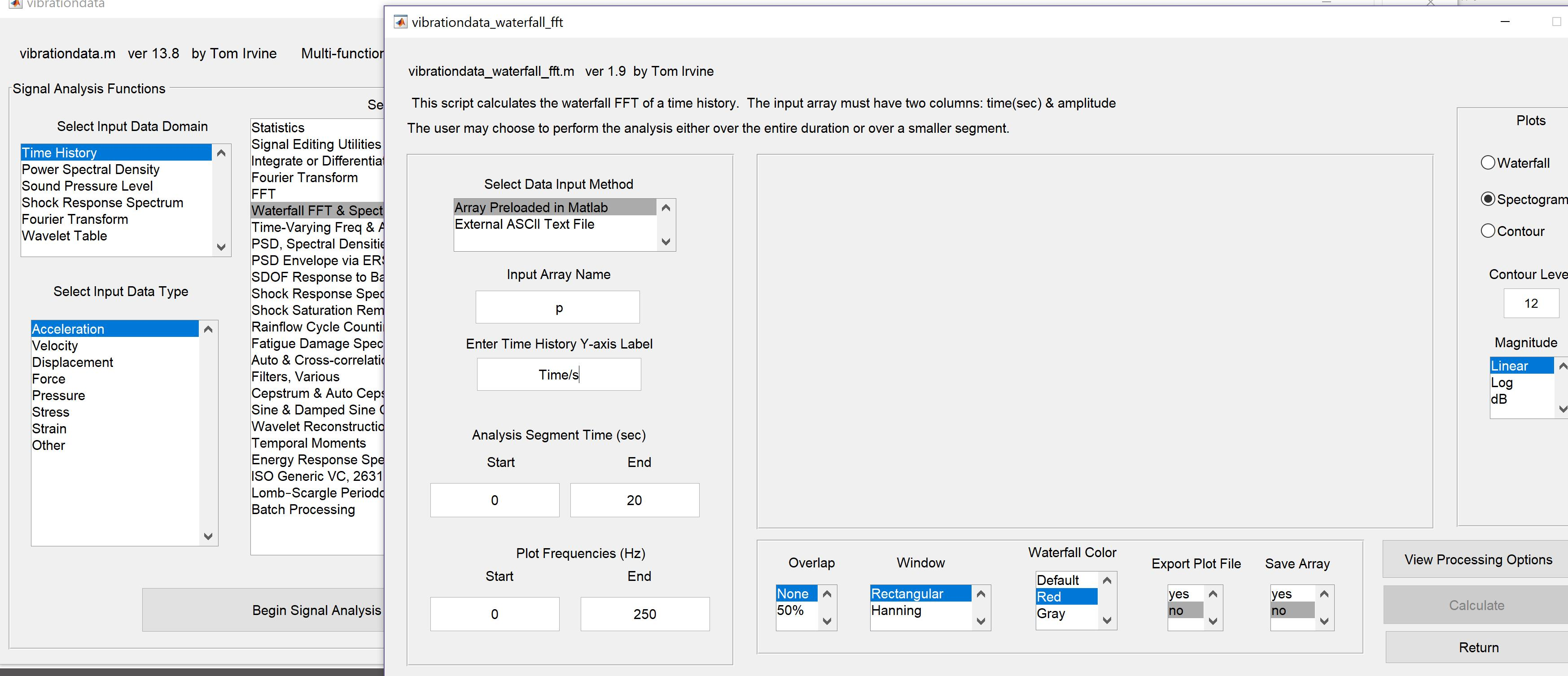Thanks very much

Xiaofu Li

•peteroznewman
Subscriber
•xiaofuli
Subscriber

1. Thanks very much for your help, I got the Waterfall FFT and Spectrogram.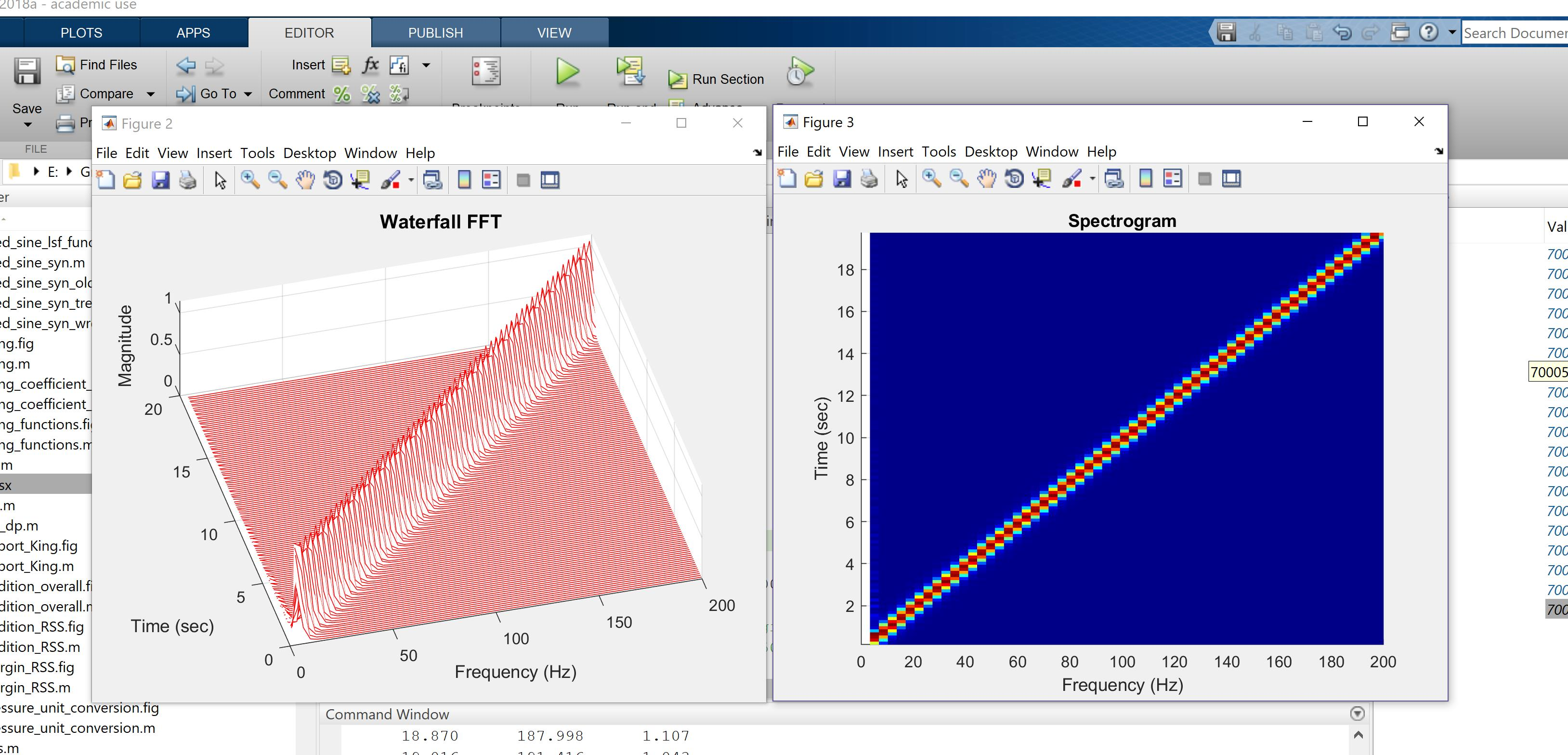2. I attached a now Workbench file, I make the highest frequency 200Hz and assign it a 80000 data points, while I still have some errors that I failed to run for 2 times, I make some change for the number of the elements within mesh generation and give a less time step, but it does not work, I hope that you could give me some suggestion or help.

3. As far as I know that we could choose the type of the element as either beam element, solid element, or shell element in APDL, while I could not find a way to assign a specific element type in workbench, I know we could pick the order of the element as linear or quadratic and make it Tet or Hex elements. Do you have some advice for that why there is no setting for element type in Workbench?

•peteroznewman
Subscriber

I downloaded your model. I made one change, I made the time step 0.00025 s (4kHz) instead of 0.0004 s and started it running. It ran for 15.4 hours on 15 cores and finished without error.

•     >>> SOLUTION CONVERGED AFTER EQUILIBRIUM ITERATION   3

•  *** LOAD STEP     1   SUBSTEP 80001  COMPLETED.    CUM ITER = 180898

•  *** TIME =   20.0001         TIME INC =  0.195001E-03

•  *** RESPONSE FREQ =  253.8       PERIOD=  0.3940E-02  PTS/CYC =  20.

•  *** ANSYS BINARY FILE STATISTICS

•   BUFFER SIZE USED= 16384

•         1.562 MB WRITTEN ON ELEMENT SAVED DATA FILE: file0.esav

•         0.125 MB WRITTEN ON ASSEMBLED MATRIX FILE: file0.full

•     10148.250 MB WRITTEN ON RESULTS FILE: file0.rst

It will take some time to look at the results.

Regards,
Peter

•xiaofuli
Subscriber

1. I will try with it as soon as possible.

2. If you take a look at the Solver.out file, you will find out that there are only 3 warning messages without any error, but it just stop, from what you have suggested that I only need to shorten the time step then I will be able to shoot for the results. Do you know why the warning along will stop the program running?

3. As far as I know that we could choose the type of the element as either beam element, solid element, or shell element in APDL, while I could not find a way to assign a specific element type in workbench, I know we could pick the order of the element as linear or quadratic and make it Tet or Hex elements. Do you have some advice for that why there is no setting for element type in Workbench?

•peteroznewman
Subscriber

3. Here is how you set an element type.

•peteroznewman
Subscriber
•xiaofuli
Subscriber

Thanks very much for your help

1.After I change the time step from 0.0004s to 0.00025s, It still end up with some errors, moreover, The solution seems fully completed, Just the post processor seems does not work very well, and I checked the solve.out file, there is no any errors except 3 warning messages. besides from the information of the solve.out, It finish all the 20s successfully, The thing is that there is just no any results shown within Workbench. I attached both the Workbench file and the solve.out file. Hope that you could help me to find out the reason.

2. One more issue is that, as shown in the following figure, The solution,as marked by a red circle, seems done completely, but from the convergence

graph, it only finish 12.7s out of 20s, I check the solve.out file, It does finish 20s. but I just have no idea why they are different?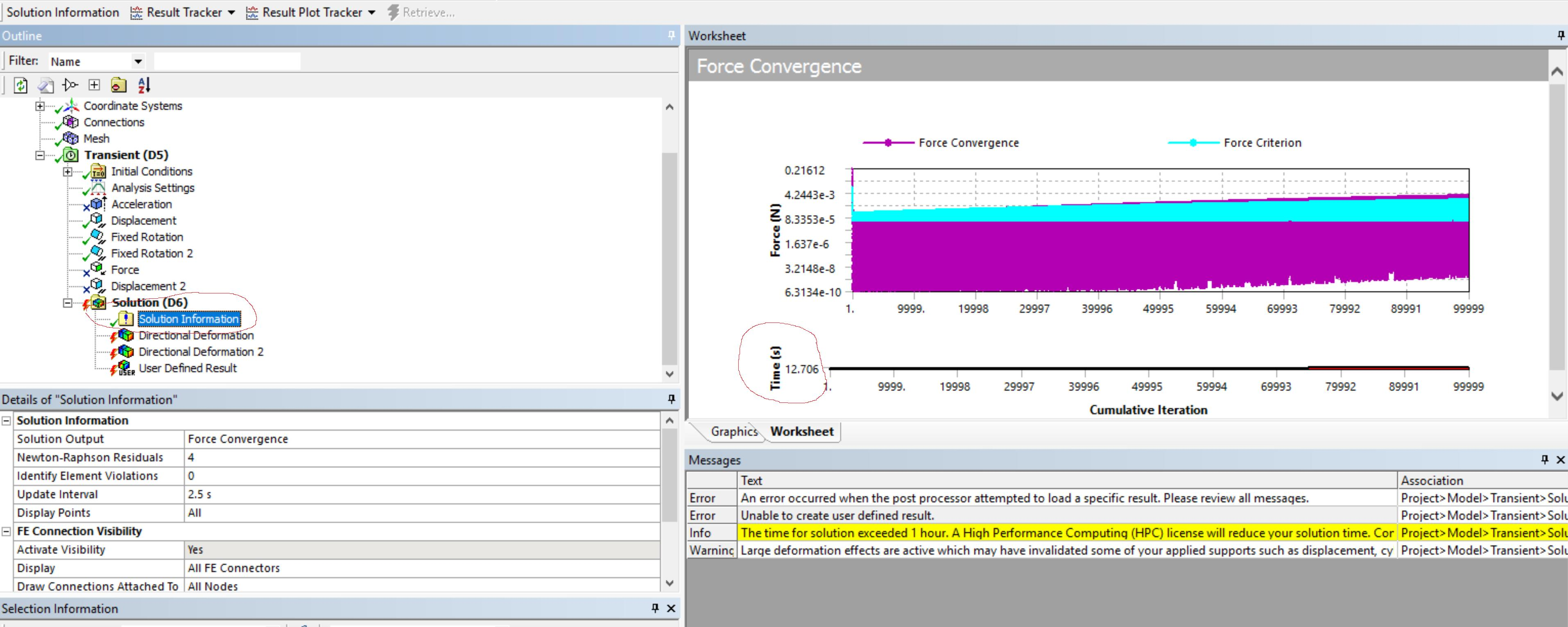Thanks

Best Regards

•peteroznewman
Subscriber

Xiaofu Li,

I experienced the same behavior in Mechanical that you did. I don't think the Mechanical program can properly display the convergence plot with 189,000 iterations, however, the solver did complete the solution and I was able, with much patience, to copy the 80,000 rows of displacement data out of a Tabular output and paste it into Excel. I have attached that file below. The displacement is one corner of the IC. I subtracted the two outputs in matlab.

Regards,
Peter

•xiaofuli
Subscriber

1. From what you stated, which means that there is actually no any solutions that could remedy my problem as long as the iterations is beyond 80000? If it is that case, what is your suggestion that could solve the issue alternatively with the highest frequency of 200Hz which need at least 80000 data points?

2. The Excel file you uploaded seems that it is just the displacement input, I think it is neither the out displacement or the IC corner nor the displacement response of the relative displacement. Could you please check it out? Thanks

Best Regards

•peteroznewman
Subscriber

1. The solver completed all 80000 time steps. All the data is available. Mechanical just couldn't display the 189,000 iterations on the convergence plot. You don't need to see the convergence plot. The solution completed without error. Forget about it.

2. The Excel file has three tabs, take another look.

Regards,
Peter

•xiaofuli
Subscriber

1. I got your point of the display of the convergence plot, while the issue is that the solver completed all 80000 time steps, All the response should be available. But I just could not get results at all, as the figure shown below

•xiaofuli
Subscriber

1. As far as I know that the linear analysis of the full transient structural is based on the equation of

"M*d^2x/dt^2+C*dx/dt+K*x=F"

"M*d^2x/dt^2+C*dx/dt+K1*x+K3*x^3=F"

While the nonlinear structural analysis will be a equation like Duffing Oscillator as shown above with one more term added as the K3*X^3, if the K3 is positive, then It will be the hardening case. Therefore, If I turn on the large deflection button, what kind of equations will the ANSYS solver use? Will it use a equation similar to the above one including the term of K3*x^3? Could you recommend some detailed information of what kind of equations will ANSYS use for large deflection case?

Thanks

Best Regards

•peteroznewman
Subscriber

1. The linear analysis is indeed the first equation you show.  The nonlinear equation is the same but K = K(x), that means the stiffness is a function of deformation. In the linear analysis, the stiffness is calculated once at the start and is constant.

There is no explicit x^3 term. It occurs more organically. The value of K(x) is computed from the element assembly after the nodes have shifted to their deformed locations. The reason the full nonlinear solution takes so long is it has to keep assembling new stiffness matrices and iterate to find the value of x that balances the forces.

The figure you are showing is the convergence plot. Stop looking at that, it's broken. Go to a Result plot like Displacement. It takes a long time, but the plot will eventually be rendered. RMB on the Tabular data, Select All, then Ctrl-C to copy.  Wait, then wait some more, then wait even longer. Eventually, it will put all that data on the Clipboard and you can then Paste it into Excel.

Regards,
Peter

•xiaofuli
Subscriber

1. Got the point of the stiffness matrices.

2. Regardless of the convergence plot, I did check the result plot like the displacement response, while the problem is that I could not get anything from the tabular data output window on the right side. The below is a new model that I run, Just change the thickness of the PCB and make the natural frequency decrease to 47Hz in order to give less data points. But the consequence is that I still fail to obtain any result, As you could see that there are red-colored lightning symbols before the output, which means it fail to get the results(As I marked). I have no idea how to solve this issue, could you please give me some suggestion?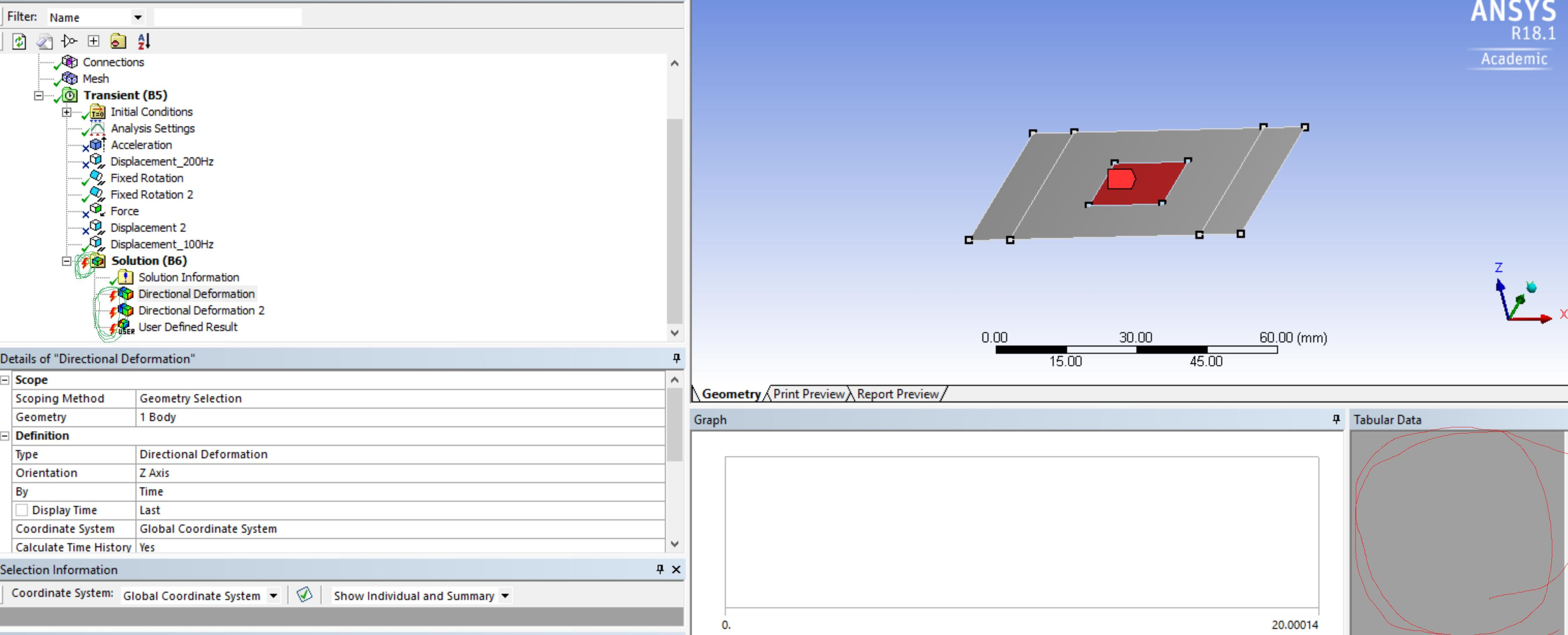Thanks

Best Regards

•xiaofuli
Subscriber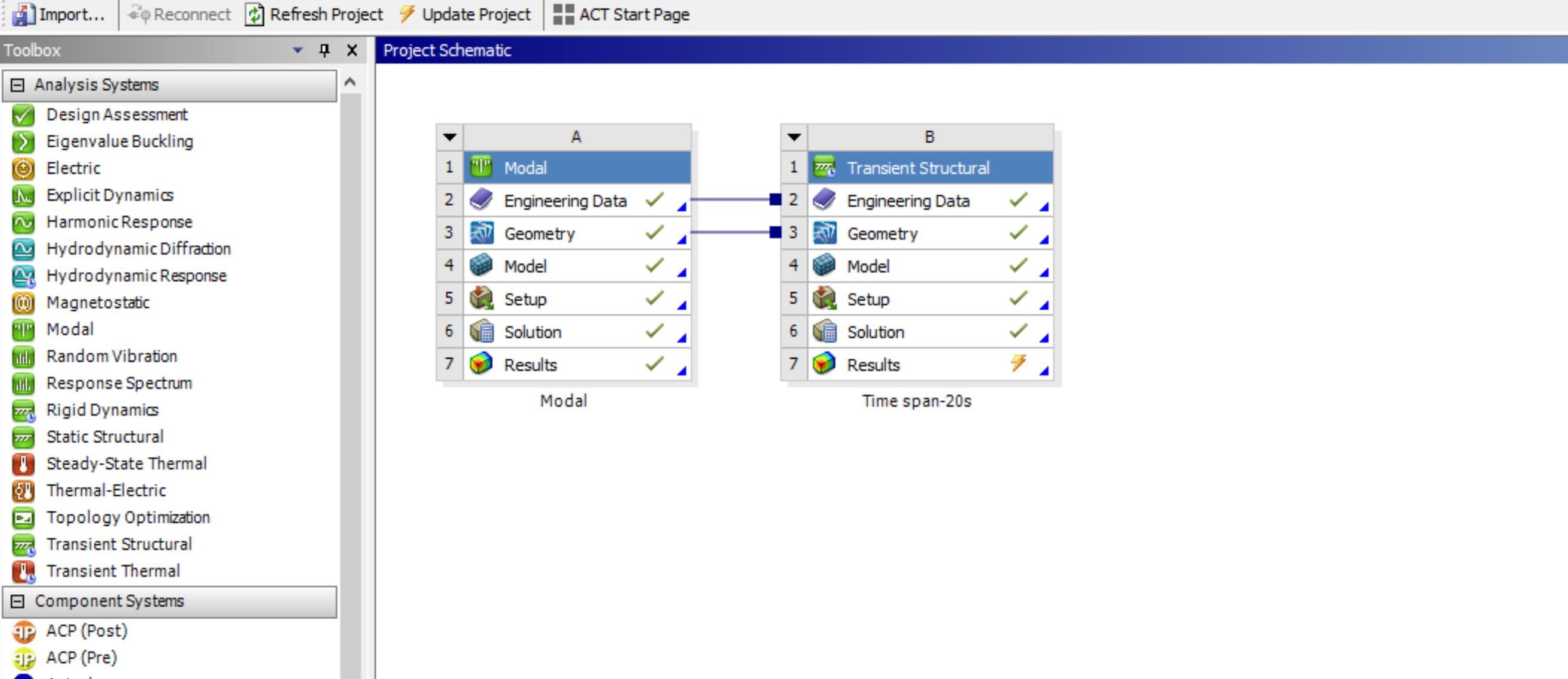The above figure shows that the solution is done, just could not load the results. The following figure is the error message, besides I check the solve.out file, no any error happens. Therefore, I kind of feel confused now. Hope that you could help me with that, I also attach the workbench file with it.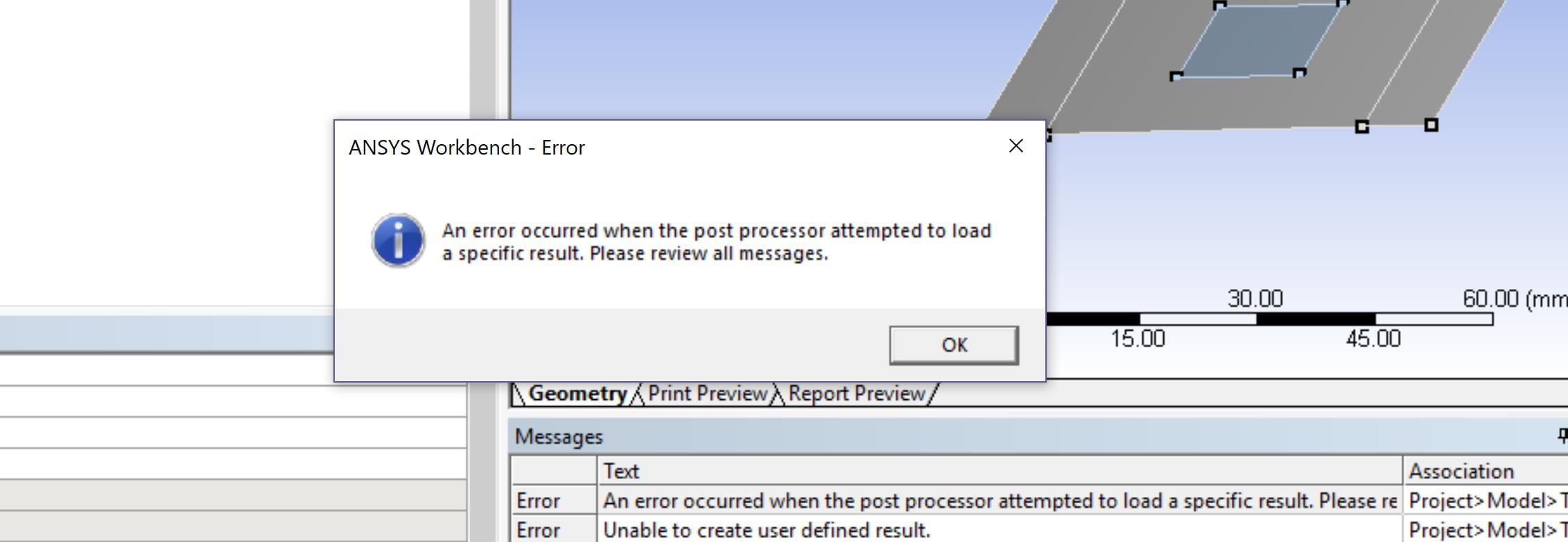Thanks

•peteroznewman
Subscriber

Try deleting the old result plot requests that have specific times saved in them and create new result plot requests. That might help.

•xiaofuli
Subscriber

Thanks very much for your help, just a few questions need to make sure.

1. will ANSYS Workbench automatically choose the accurate element type to analyze the nonlinear large deflection problems?

2. Is there any way that i could add some equations of motions by using the command within ANSYS Workbench?

Thanks

•peteroznewman
Subscriber

1. There are many options to set on a standard element and ANSYS automatically sets the best option for each problem, but you can override that setting if you want.

2. A textbook, single DOF equation of motion solution can be simulated by assembling a mass on a translation joint and a spring and damper. The equations of motion are written by ANSYS for that model and then solved.